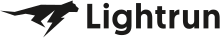• Dev Observability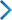• Product• Pricing
• Docs
• Resources• Blog
• Company• Debug Wordle# Stuck on an issue?

Lightrun Answers was designed to reduce the constant googling that comes with debugging 3rd party libraries. It collects links to all the places you might be looking at while hunting down a tough bug.

And, if you’re still stuck at the end, we’re happy to hop on a call to see how we can help out.

## Write a Python program to convert decimal to binary

See original GitHub issue

### Description

Write a Python program to convert decimal to binary

Binary is a base-2 number system. It uses only two digits: 0 and 1. The digits are called bits. A bit is the smallest unit of information in a computer. A bit can be either 0 or 1. A bit can be used to represent a single value, such as 0 or 1, or it can be used to represent a single state, such as on or off.

To convert a decimal number to binary, we can use the following algorithm:

1. Divide the decimal number by 2.
2. Write down the remainder.
3. Divide the quotient by 2.
4. Write down the remainder.
5. Repeat steps 3 and 4 until the quotient is 0.
6. The binary number is the sequence of remainders written in reverse order.
``````Input  : 10
Output : 1010
``````
How to contribute
• Save the solution in `program/convert-decimal-to-binary.py` file
• Add `convert-decimal-to-binary.py` file in `convert-decimal-to-binary` folder

### Issue Analytics

• State: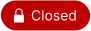• Created 4 months ago

####Top GitHub Comments

1reaction
harshraj8843commented, Oct 14, 2022

Hey @harshhes

You can comment `!assign` on any `good first issue` you want to contribute to, to be auto-assigned

``````!assign
``````
0reactions
harshraj8843commented, Oct 14, 2022

Hey @harshhes, this issue is already assigned to @olutofunmi !!!

Thanks for your interest in contributing to this project.

####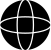Top Results From Across the Web

Python program to convert decimal to binary number
Given a decimal number as input, the task is to write a Python program to convert the given decimal number into an equivalent...
Python Program to Convert Decimal to Binary - BeginnersBook
In this program we have defined a function decimalToBinary() for the conversion. This function takes the decimal number as an input parameter and...
Python Program to Convert Decimal to Binary ... - Programiz
Decimal number is converted into binary by dividing the number successively by 2 and printing the remainder in reverse order. How to convert...
Convert Decimal to Binary in Python [3 Methods]
Convert Decimal to Binary in Python using While Loop ... In this method, we accept the decimal number as an input and divide...
Convert Decimal to Binary in Python with Example Program
In Python, we can simply use the bin() function to convert from a decimal value to its corresponding binary value. The bin() takes...

####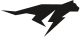Troubleshoot Live Code

Lightrun enables developers to add logs, metrics and snapshots to live code - no restarts or redeploys required.
Start Free

####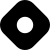Top Related Hashnode Post